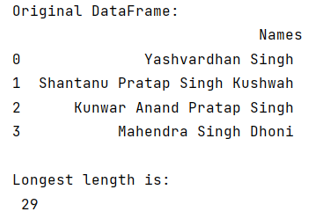# Find length of longest string in Pandas DataFrame column

Given a DataFrame, we need to find length of longest string in pandas DataFrame column.
Submitted by Pranit Sharma, on July 23, 2022

## Pandas DataFrame

Pandas is a special tool that allows us to perform complex manipulations of data effectively and efficiently. Inside pandas, we mostly deal with a dataset in the form of DataFrame. DataFrames are 2-dimensional data structures in pandas. DataFrames consist of rows, columns, and data.

## String

The string is a group of characters, these characters may consist of all the lower case, upper case, and special characters present on the keyboard of a computer system. A string is a data type and the number of characters in a string is known as the length of the string. For example, if a string consists of 5 letters, the length of that string will be 5.

## Find length of longest string in Pandas DataFrame column

To find the length of the longest string in a column of pandas DataFrame, we are going to use str.len() method which will return the length of each string, and then we will use max() function which will return the longest length among all the lengths.

Note

To work with pandas, we need to import pandas package first, below is the syntax:

```import pandas as pd
```

Let us understand with the help of an example,

## Python program to find length of longest string in Pandas DataFrame column

```# Importing pandas package
import pandas as pd

# Creating a Dictionary
d = {'Names':['Yashvardhan Singh','Shantanu Pratap Singh Kushwah','Kunwar Anand Pratap Singh','Mahendra Singh Dhoni']}

# Creating a DataFrame
df = pd.DataFrame(d)

# Display original DataFrame
print("Original DataFrame:\n",df,"\n")

# Returning the longest length
print("Longest length is:\n",df.Names.str.len().max())
```

## Output